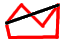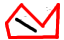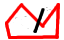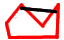# Know if an edge is inside or outside a polygon

Hi, I already made a function to know if a vector crosses any edge and now I need to test if the vector is inside or outside a polygon.I want to create edges inside the polygon.

Example: The only valid edge starting in the white vertex ends with the light blue vertex.

My algorithm already excludes all other verts but the red one.

How do I know if the generated edge is inside the polygon?

suppose you have an edge [AB]
first test if A is inside the poly,
then test if [AB] intersect with any of the polygon segment.
here is a function to test if a point is inside a poly (from oce) :

``````def pointInPoly(x,y,poly):
inside =False
p1x,p1y,z = poly
n = len(poly)
for i in range(n+1):
p2x,p2y,z = poly[i % n]
if y &gt; min(p1y,p2y):
if y &lt;= max(p1y,p2y):
if x &lt;= max(p1x,p2x):
if p1y != p2y:
xinters = (y-p1y)*(p2x-p1x)/(p2y-p1y)+p1x
if p1x == p2x or x &lt;= xinters:
inside = not inside
p1x,p1y = p2x,p2y
return inside
``````

x and y are coordinates
poly is a list of coords that represents the polygon perimeter.
returns true if inside
hope this can help

The edges / vectors only utilize the vertices of the polygon so the points should they be considered inside or outside the polygon?

Example:
For the white vert my function returns as result the red and the light blue. The function doesn’t discriminate if the edge is outside the polygon but already calculates intersection of other edges with this edge and thus eliminates those results.

so i have:

• edge(white,red) # this is the edge that is causing problems
• edge(white,light_blue) # accurate

after running my function i know that the edges returned do not intersect with any other edge of the polygon.

How do I know if the first edge is outside the polygon?

first test if A is inside the poly,
then test if [AB] intersect with any of the polygon segment.
These 2 points are already true right (because the results were already filtered by the previous function)? I don’t understand the next steps howeverSorry for being lost :s

You could test the midpoint (or a number of sub points) of the newly created edge to ensure that its within the polygon.

The thing is i need performance badly

EDIT: I’ve seen a pretty interesting solution but I don’t know how to do this:
render the plane in white (any color different from the background) and create a transformation matrix and use that image in conjunction with the coordinates and the matrix to know if a vert of (x,y,z) --> matrix --> (u,v) coordinates is white and thus inside or outside a plane. Can someone do this?

Ok, the reason why I’m doing all this is because Blender.Geometry.Polyfill() isn’t working for me with the above polygon. It creates faces with the (white,green,red) and the (red,purple_blue,yellow)

There is an algorithm that tests if any point is inside a polygon by counting the number of inersections from the tested vertex and out in the infinite. You can find it at several places on the web. It is as quick as it can get and easy to implement and works for convex or concave polygons.

You can use this algorithm on your problem: Suppose you drawn an edge from the white to red vertices. Then the green vertex would be detected as being inside the white-red-blue-yellow-cyan-orange-white poly. This can be generalized for any number of skipped vertices.

I read and re-read this post, and I’m not sure I am getting what you are looking for. I’m kinda dense like that. But I wrote my own modelling software a few years ago before I discovered Blender, and this guy helped me out tremendously.

I needed collision detection for …um…collision detection…and to perform boolean operations, and I wasn’t far enough in my math classes in college to know the math I needed. This guy is a genius. Study some of it, I hope it helps.

http://local.wasp.uwa.edu.au/~pbourke/geometry/

the midpoint test won’t work (alone) in all cases, as you can have a part of a segment inside the poly and the other part outside.ok I get it, your points are the verts of the poly itself. so you assume they are in the poly.
but the function above will return false if the point is on the perimeter (your case)

if 2 points A and B are (really) inside a poly, one can tell that if the segment [AB] do not cross any segment of the polygon, [AB] is completely inside :

no intersections (inside)one intersection (goes outside)in your case (it’s also mine for the city engine) I use one of the 2 tricks :
either I enlarge the poly,
either I do the test with point slightly inside the poly :
rather than test this :I test this :so it’s like the common case.

the thing is to find the correct location for the 2 test points :

``````
from Blender.Mathutils import *

def angleEnlarge(c0,c1,c2,w) :
c0=Vector(c0)
c1=Vector(c1)
c2=Vector(c2)

v0=c1-c0
v1=c2-c1

b=writeVec(w,rot-90)
b=b+c0
c=b+v0

d=writeVec(w,rot-90)
d=d+c1
e=d+v1

interlist=LineIntersect(b,c,d,e)
if type(interlist)==type(tuple()) :
return interlist
else : # c0 c1 c2 are on the same line
return c
``````

c0, c1 and c2 are 3 points of the poly, [x,y,z] or Vector[x,y,z]. z does not matter.
must be listed in anti-clockwise order otherwise you’ll obtain the outside points
w is the offseted point of c1. try 0.01 for example.
w positive is inside, w negative is outside (when anti-clockwise list)

here two other functions it needs to compute vectors :

``````def readVec(side) :
a=side
c=side
try :lenght=sqrt((a*a)+(c*c))
except :
if str(a)=='-1.#IND' : a=0
if str(c)=='-1.#IND' : c=0
lenght=0
rot=atan2( c, a )*(180/pi)
return lenght,rot

def writeVec(lenght,alpha,z=0) :
x=(lenght * sin(beta)) / sin(alpha+beta)
y=(lenght * sin(alpha)) / sin(alpha+beta)
return Vector([x,y,z])
``````

readVec takes a vector like [1,2,0] (x+1 and y+2) and returns a lenght and a degree
writeVec takes a lenght and a degree and returns a vector

I use these functions in oce, these one are a bit simplified but you can find them in /bin/primitives.py

it’s a bit tedious I knowmaybe there’s something better but I don’t find it.
you’ll also have to convert your poly as a list of verts coordinates like :

``````
poly=[[0,0,0],[1,0,0],[1,1,0],[0.7,1,0],[0.5,1.3,0],[0.3,1,0],[0,1,0]]

``````

I edited the pointinpoly function, there was a call to another function, useless here.

@littleneo, I was under the impression, based on his earlier post, that he already had an algorithm that excluded those cases where the new edge crossed over existing edges of the polygon. In such a case, the midpoint way will work because he’s only got inside or outside edges, i.e. no new edge he’s generating will cross over another one (assuming his current algorithm works properly).

So my guess is you’re really trying to rewrite the fill function, correct?

1- i’ll try to redo the algo given here
and be back with any questions if problems !

2

the bourke site has this math intro for point inside polygone

http://local.wasp.uwa.edu.au/~pbourke/geometry/insidepoly/

it’s tedious but it’s math what can it say!

the second solution with sum of angle = 2 pi or 0 seems tinteresting enough and simple
but the C code is difficult to read and translate !

unless someone else know how to do that !

let us know if you cn translate that in python

hope it helps

thanks

Hi, Alberto & Others!

I think we discussed the issue 2-3 months ago. I also provided a solution dealing with that sort of issues. I can provide links if you cannot find it yourself before that(Sorry, I dont have time to search the forum right now)

Sorry, I havent read entirely the points raised earlier in this thread + the code, so there MAY appear some repetition, ok? I just wanna pose some points that solve the issue…

At first, Alberto - YES, polyfill() works badly with that type of polygons! My solution covered exactly this (as far as I remember). The key is to calculate correctly internal angles of the polygon. It is NOT possible with current API function cause you always get an angle between 0-180 degrees. If your internal angles are ALL < 180 degrees, then polyfill() or other custom proc works correctly & nice. The problem comes when you have internal angle > 180 as it is at the GREEN vertex. Sooo when reaching such a vertex (like the green one) the proc to generate internal edges should do something… My suggestion was to shift the first vertex from WHITE to exactly the GREEN one… until another such case is met while trying to connect other verts, ok? :spin: Im not quite sure if I published this proc (or it is inside one of my modules) but I am sure I have published NOW TO calculate the real angles, including internal ones as in our case…

There is a second tactics coming from the Math (which sound easy but I havent implemented so far in Python)… The point is exactly answer to main question of Alberto - How to determine that one point (vector) is inside a polygon or outside. It is really a problem to know if you’re inside or outside a figure… It seems that there is NO stable criterion on that… But I’ve read an article saying that if you look at a ray starting from that point of interest and going endlessly far away at a certain direction (say parallel to X axis), then the number of crossings with your polygon of interest gives you a test to answer the question your point is inside or outside the polygon. Should that number is odd => the point is inside and should the number is even => your point is outside, ok?The polygon should be a closed one, not an open polyline as the math issue is NOT TRUE in that case… And it is natural cause there is NO inside or outside in the case of open polylineHope this helps! :eek:

Regards,

may be Abidos has a good solution too here ?
but cant put my finger on the thread!

from Bourke site

the second solution seems to be good

the second solution with sum of angle = 2 pi or 0 seems tinteresting enough and simple
but the C code is difficult to read and translate for me at least !

sorry but almost never work with C before

unless someone else knows how to do that !

let us know if you can translate that in python

Thanks

yep you’re right forTe the midway point test can be useful if some cases have been tested first, that’s why I wrote ‘(alone)’.
abidos gives a great trick about the odd/even thing. supposing you know if a starting point is in or out of a shape, you can determine where the other are by testing the segment intersections, and remember the in/out status of the last tested point.

ps ricky : my pointinpoly function is a python translation of the first c codeWell said littleneo.

I wish wish wish I could write Python script! I’d help you here!

The concept, mathematically, leads to ambiguity in the starting point of your vector, that is, whether your starting point is inside the polygon or not.

Otherwise, the mathematics are designed to return the precise points where
the vector is inside the polygon. Since you only need to know if it’s inside or not, you don’t need to look too hard at the equations. you only need to calculate mu, as it will return a value >=0 and <=1 for your vector to lie inside the poly. DotProduct and CrossProduct are your important functions.

i think the second way to bourke seems very simple
just calculate s the angle for each verte and sum

i think this should a sinpler algo!

would be nice to see an implementation in python for this one!

i’l try to make the algo works with the first way of doing it

happy blendering

Hiya,

I published here the implementation of the first possible solution I described in my yesterday’s post under this thread… It works quite straight-forward considering each time to add new triangle face by checking if the three vertices invoved make a left-handed triple or not. If “YES” => the new edge to add (and needed for constructing the new face) is completely INSIDE the contour :spin:

Regards,

Abidos
by adding a circle with 7 sides selected it and then run your script but it did not do any faces in edit or object mode !

can you explain why it is not doing it ?

thanks

Well, Ricky this is a good point but please ask such things in the Cover contours script’s thread here so that people know the bugs and possible solution… Pls find your answer in the other threadFirst of all thanks to everyone@Abidos : what do you mean with right-handed triplets?

@Everyone: i had a lines_intersect() that seems sluggish after seeing this: http://local.wasp.uwa.edu.au/~pbourke/geometry/, however, it works for 3D and that link seems to be 2D.
My question is: Do we throw away the z because all lines are in the same plane?
And what if the plane was perpendicular to y? (Vertical)?
I also heard of throwing away the biggest component in the plane normal and using the other 2.

But anyway here it is ( create a quad and play with the vert positions and see the intersection point change as you re-run the script):

``````import Blender
from Blender import Object
from math import *
Blender.Window.EditMode(0)
me = Object.GetSelected().getData(mesh=1)

def vectorize(v1,v2): #CORRECT ORDER
"""Creates a vector from 2 3D points"""
return [v2-v1,v2-v1,v2-v1]

class Vector:
def __init__(self,co=[0,0,0]):
self.co = co

def co(self):
return self.co

self.co = [self.co+vec,self.co+vec,self.co+vec]
return self.co
def __sub__(self,vec):
self.co = [self.co-vec,self.co-vec,self.co-vec]
return self.co
def __mul__(self,vec):
self.co = [self.co*vec,self.co*vec,self.co*vec]
return self.co
def __div__(self,vec):
print "fix me!"# not working with modulo
self.co = [float(self.co/vec),float(self.co/vec),float(self.co/vec)]
return self.co

def len(self):
import math
return  math.sqrt(self.co*self.co + self.co*self.co + self.co*self.co)

def scale(self,value):
self.co = [self.co*value,self.co*value,self.co*value]
return self.co

def parallelity(self,vec2):
"""Returns a percentage of parallelism between vectors in decimal form.

The abs() of the number returned:
1 means 100% parallel
values in between also work
0 means 0% parallel (perpendicular vectors)

The sign of the value returned is the orientation between 2 vecs.
If the vecs form an angle under 90º the value is negative and vice-versa.

Usage:
# Because of floating-point precision use 5 decimal places instead of an integer to test parallelism
if abs(parallel_vecs(vec1,vec2)) &gt; 0.99999:
print "vecs are 100% parallel"
if abs(parallel_vecs(vec1,vec2)) &lt; 0.00001:
print "vecs are 100% perpendicular"
if parallel_vecs(vec1,vec2) &gt; 0:
print "vecs form an interior angle bigger than 90º"
angle = math.acos(parallel_vecs(vec1,vec2))
"""
mod = self.len() * Vector(vec2).len()
if mod == 0: return 0
return (self.co*vec2+self.co*vec2+self.co*vec2)/mod

def reverse(self):
self.co = [-self.co,-self.co,-self.co]
return self.co

def angle(self,vec):
# Unfortunately ( try to fix ), even if par appears to be 1.0 due to floating point precision you must set it to 1 to avoid MATH DOMAIN ERROR ( same applies for -1.0 )
from math import acos
par = self.parallelity(vec)
if par &gt; 0.999: return 0
if par &lt; -0.999: return 3.14159
return acos(par)

def lines_intersect(me,ray,edge):
"""Finds the intersection of an incident vector (ray) and the edge and returns k
P is the point of intersection of the 2 vectors.

P = edge + (Q1-Q0) * k

^^^^^^^^^^^^^^^Google parametric equations if you don't understand^^^^^^^^

If 0&lt;k&lt;1: ray intersects the edge
If k&lt;0 or k&gt;1: intersection occurs outside the edge
If k is None: no intersection is possible
"""
global Q0,Q1
Q0 = me.verts[edge].co
Q1 = me.verts[edge].co

u = Vector(vectorize(me.verts[ray].co,me.verts[ray].co))
v = Vector(vectorize(Q0,Q1))
parallelity = u.parallelity(v.co)

# If the function is not returning k even when desired change the treshold below:
print ray
print edge

if abs(parallelity) &gt; 0.999: # ray and edge are parallel
if ray in edge or ray in edge: return "Coincident"
else: return None

if ray == edge: return 0 # the edges are not parallel so intersect at the origin

base = Vector(vectorize(me.verts[ray].co,Q0))
base2 = Vector(base.co).reverse() # use for v to fit vector starting points

#c is the base vector ( ray,Q0 )
uc = u.angle(base.co)
vc = v.angle(base2)

tan_uc = tan(uc) # calculated earlier for speed purposes
k_num = base.len() * tan_uc
k_den = v.len() * cos(vc) * ( tan_uc + tan(vc) )

k = k_num/k_den

P = Vector(Q0) + Vector(vectorize(Q0,Q1)).scale(k)

#############  TRY TO MAKE THIS FASTER BY CREATING A MORE EFFICIENT VALIDITY TEST
############# THAT DOES NOT REQUIRE RECALTULATING K AND THE POINT OF INTERSECTION P

if k &lt; 0.999 and k &gt; 0.001 and abs(u.parallelity(vectorize(me.verts[ray].co,P))) &lt; 0.99: # k&lt;0.999 avoids bugs as the vector is almost null and the angle will be wrong, k&gt;0.001 doesn't avoid bugs but avoids unnecessary calculations
print "WARNING: FLIPPED VECTORS --&gt; FIXING"
uc = pi - uc # vector crossed base to the wrong side # why does it work with any k*pi,k=Integer number?

tan_uc = tan(uc) # calculated earlier for speed purposes
k_num = base.len() * tan_uc
k_den = v.len() * cos(vc) * ( tan_uc + tan(vc) )

k = k_num/k_den

return k

poly_len = 4 # len of verts in your poly ( excluding the new intersection point)
ray = (1,2)
edge = (0,3)

k = lines_intersect(me,ray,edge)

print "L",len(me.verts)
try:me.verts.delete(range(poly_len,len(me.verts)))
except:pass

if k &lt; 1 and k &gt; 0: print "intersect"
print k

if k is not None and k is not "Coincident":
P = Vector(Q0) + Vector(vectorize(Q0,Q1)).scale(k)
print "K",k
print P

me.verts.extend([P])
me.edges.extend([(ray,len(me.verts)-1),(edge,len(me.verts)-1)])
else:print "K is none"
me.sel=0
me.verts.sel=1
Blender.Window.EditMode(1)
``````